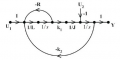# Transfer function from signal flow graph

#### ansh_kumar

Joined Jun 24, 2013
3
In the system whose signal flow graph is shown in figure. $$U_1(s)$$ and $$U_2(s)$$ are inputs. The transfer function $$\frac{Y(s)}{U_1(s)}$$ is1. $$\frac{k_1}{JLs^2 + JRs + k_1 k_2}$$
2. $$\frac{k_1}{JLs^2 - JRs - k_1 k_2}$$
3. $$\frac{k_1 - U_2(R + sL)}{JLs^2 + (JR -U_2L)s + k_1 k_2 - U_2R}$$
4. $$\frac{k_1 - U_2(R - sL)}{JLs^2 - (JR -U_2L)s - k_1 k_2 + U_2R}$$

I have a doubt that whether the input $$U_2$$ will come in the transfer function or not? I'm confused between option (1) and (3).

#### WBahn

Joined Mar 31, 2012
26,062
Do the math. Show your work to determine Y(s) as a function of U1 and U2 and then see what you are left with after dividing by U1.

#### MrAl

Joined Jun 17, 2014
7,756
In the system whose signal flow graph is shown in figure. $$U_1(s)$$ and $$U_2(s)$$ are inputs. The transfer function $$\frac{Y(s)}{U_1(s)}$$ is
View attachment 120607
1. $$\frac{k_1}{JLs^2 + JRs + k_1 k_2}$$
2. $$\frac{k_1}{JLs^2 - JRs - k_1 k_2}$$
3. $$\frac{k_1 - U_2(R + sL)}{JLs^2 + (JR -U_2L)s + k_1 k_2 - U_2R}$$
4. $$\frac{k_1 - U_2(R - sL)}{JLs^2 - (JR -U_2L)s - k_1 k_2 + U_2R}$$

I have a doubt that whether the input $$U_2$$ will come in the transfer function or not? I'm confused between option (1) and (3).
Hello there,

Did you find the complete transfer function first? Once you find the complete transfer function it will become obvious which one is right if this is a multiple choice question. There will be only one right answer, period, and you wont get two answers just because you consider U2 to be part of the whole transfer function or not, three will be just wrong no matter what you do as long as you do the math correctly. I dont want to say anything too much more yet because i'll give it away